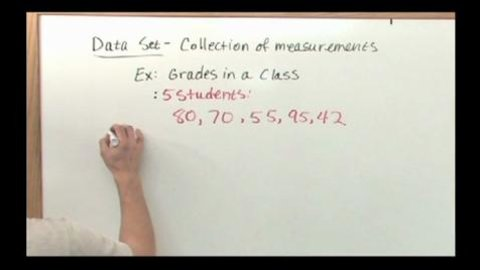# Mean, Median and Mode Part of the Series: Probability and Statistics Tutor Series

##### TMW Media## Related videos

Mean & Standard Deviation of the Binomial Distribution
This program covers the important topic of the Mean and Standard Deviation of the Binomial Distribution in Probability and Statistics. We begin by discussing the Binomial Distribution and its mean. Next, we solve several problems that involve the Mean and Standard Deviation of this important distribution to give students practice…
Math Series 1
A math series designed for grades 7th to 9th to help students learn and understand math concepts.
Algebra: A Piece of Cake
Covers variable, numerical substitution, algebraic conventions, developing algebraic formulas from number patterns.
Factoring is Fantastic
Key concepts explained: Factoring, terms, expressions, finding the greatest common factor(s), and factoring polynomial expressions that are the sums or differences of terms with common factors. This starts with a review of numerical factors and greatest common factors, and moves into the realm of algebra. Different types of factoring and…
Integer Operations into Negative Zone
Key concepts explained: negative numbers, integers, addition and subtraction of integers.
Linear Equations and Their Graphs
Key concepts explained: linear equations and their graphs, and the domain of an equation.
Percentages: That Make Sense
Part of the Series: Math Series 1
Key concepts explained: converting percentages into decimals and fractions, and converting decimals and fractions into percentages. Taking examples from the widespread use of percentages in everyday life from the percentage of cappuchinos to regular coffee a coffee shop sells, to the percentage of sharks to other fish in an aquarium,…
Slopes: That's a Little bit Steep
Part of the Series: Math Series 1
Key concepts explained: horizontal and vertical lines, positive and negative slopes, calculating a slope by measuring its rise and run, and calculating a slope by using two points on a coordinate plane.
Math Series 2
Math lessons including Geometry, Probability, Problem Solving, Trigonometry and Ratios.
Geometry
Key concepts: formulas for a parallelogram (square, rectangle, and rhombus) & formulas for a trapezoid & triangle.
Probability
Expressing a probability between certainty and impossible as the likelihood of an uncertain event occurring --conducting and recording probability experiments using small and large number trials with replacement --calculating the theoretical probability of a particular event occurring --expressing probabilities as odds
Problem Solving
The five steps to problem solving: read and re-read the problem, identify important information, choose a strategy, solve, check the answer --the six strategies to choose from: guessing and checking, using a simpler related problem, discovering patterns, drawing pictures and diagrams, making lists and charts, working backwards --the guessing and…